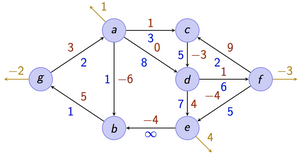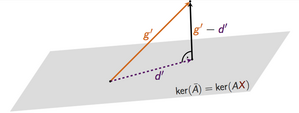# Basic Information

Lecturer: Andreas Karrenbauer Tuesday + Thursday, 14:00 - 16:00, E1.4 room 024; Maximilian John Ahmed Abbas, Sören Bund-Becker Monday, 10-12, room 024, E1 4 (except on May, 7 and May, 22: room 021, E1 4)Friday, 12-14, room 024, E1 4 9 Basics in linear algebra, discrete mathematics, calculus, algorithms, and complexity. At Saarland University these topics are covered in the bachelor courses Mathematik für Informatiker 1 & 2, Grundzüge der Theoretischen Informatik, and Grundzüge von Algorithmen und Datenstrukturen. Your final grade will be the best of the final exam and the re-exam. You may bring one A4 cheat sheet (double-sided, in your own handwriting) to the exams. Exams might be oral if there is only a small number of registered participants.Final Exam: July 27th, 13:30 - 16:30, Günther-Hotz-HörsaalRe-Exam: TBA

# Announcements

• If you want to participate in the course, please register to our mailing list!
• The tutorial assignment is finished and can be found here.
• The lectures are permanently moved to room 024 in E1.4.
• The tutorial on Pentecost Monday (21st of May) is going to be moved to Tuesday (22nd of May), same time (10-12) to room 021 in E1 4.

# Description

This course provides an introduction to fundamental concepts and algorithmic methods for solving linear and integer linear programs.

Linear optimization is a key subject in theoretical computer science. Moreover, it has many applications in practice. A lot of problems can be formulated as (integer) linear optimization problem. For example, combinatorial problems, such as shortest paths, maximum flows, maximum matchings in graphs, among others have a natural formulation as a linear (integer) optimization problem. In this course you will learn:

• how to optimize a linear function subject to linear constraints
• how to formulate combinatorial problems as (integer) linear optimization problems
• how to solve them

To this end, basic concepts from polyhedral theory will be introduced. The simplex algorithm and the ellipsoid method will be presented. The lecture concludes with exact and approximation algorithms for NP-hard optimization problems. There will be theoretical and practical exercises.# Policies

This is a 9-credit-point core lecture ("Stammvorlesung"). There will be two lectures and one exercise session per week. We will hand out exercises every week (usually worth 40 points) and each student should score at least 50% in the first half of the course (first 6 exercise sheets) and 50% in the second half in order to be allowed to take the exam.

# Lectures

The slides of all lectures condensed in a handout can be found here. The supplement containing important definitions, theorems and proofs can be found here.

DateTopicReferenceHomeworkNotes
Apr 10Overview Sheet 0, Solution 0Slides
Apr 12Polyhedra and Integer OptimizationChapter 6 in [W]; Chapter 11.8 in [BT] Slides
Apr 17Integer Optimization and ApplicationsChapter 7 in [W]; Chapter 11.2 in [BT]Sheet 1, Solution 1Slides
Apr 19Branch and Bound, DualityChapter 4.7, 11.2 in [BT] Slides
Apr 24Fourier-Motzkin Elimination, Weak/Strong DualityChapter 2.8, 4 in [BT]Sheet 2, Solution 2Slides
Apr 26Complementary Slackness, Vertices and FacetsChapter 2.6, 2.3 in [BT] Slides
May 3Existence and Optimality of Extreme PointsChapter 2.5, 2.6 in [BT]Sheet 3, Solution 3Slides
May 8Brute-force LP algorithm, degeneracy, basic directionsChapter 3.1, 3.2 in [BT]Sheet 4, Solution 4Slides
May 15The Simplex methodChapter 3 in [BT]Sheet 5, Solution 5Slides
May 17Degeneracy, Full Tableau implementationChapter 2.4, 3.3 in [BT] Slides
May 22Introduction to the Ellipsoid methodChapter 8 in [BT]Sheet 6, Solution 6Slides
May 24The Ellipsoid methodChapter 8 in [BT] Slides
May 29The Ellipsoid methodChapter 8 in [BT]Sheet 7, Solution 7Slides
Jun 5Ellipsoid - Complexity and Equivalence of Optimization/SeparationChapter 8 in [BT]Sheet 8, Solution 8Slides
Jun 7Introduction to Interior Point methodsChapter 9 in [BT] Slides
Jun 12Interior Point methods, central pathChapter 9 in [BT]Sheet9, Solution 9Slides
Jun 14Integer PolyhedraChapter 3 in [W] Slides
Jun 19Total unimodularity, Min-Cost flowChapter 3.2 in [W]Sheet10, Solution 10Slides
Jun 21Network flowsChapter 7 in [BT] Slides
Jun 26Network simplex, negative cost cycle canceling algorithmChapter 7.3, 7.4 in [BT], Chapter 3.3 in [W]Sheet 11, Solution 11Slides
Jun 28Max Flow, Min Cut, MatchingChapter 7.5 in [BT] Script
Jul 3Approximation algorithms, Matching vs Vertex CoverChapter 10.3 in [BT], Chapter 4 in [W]Sheet 12, Solution 12Script
Jul 5Video lecture on matchingChapter 8.2 in [MG]Bonus sheet
Jul 10Set cover  Script
Jul 12Video lecture on SAT problems­­  Script
Jul 17Max Cut and Semidefinite Programming  Script
Jul 19Max Cut and Semidefinite Programming  Script

# Literature

Good textbook on the topic include: